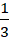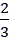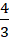# CAT 2019 Question Paper | Quants Slot 1

###### CAT Previous Year Paper | CAT Quants Questions | Question 11

A very straightforward arithmetic question similar to the ones we have seen in CAT previous year paper. It involves probably 2 concepts from Percentages & Ratio proportion. Good diligence will ensure accuracy in these questions. Going through a lot of Mechanical preparation will help you quickly solve this question & put it in your bag.

Question 11 : A person invested a total amount of Rs 15 lakh. A part of it was invested in a fixed deposit earning 6% annual interest, and the remaining amount was invested in two other deposits in the ratio 2 : 1, earning annual interest at the rates of 4% and 3%, respectively. If the total annual interest income is Rs 76000 then the amount (in Rs lakh) invested in the fixed deposit was [TITA]

## Best CAT Coaching in Chennai

#### CAT Coaching in Chennai - CAT 2022Limited Seats Available - Register Now!Let Rs x be invested in FD.
So, Remaining amount = Rs. 15 Lakhs - x, which is invested in the ratio 2 : 1
So, each investment would have(15-x) and(15-x) investments respectively
So, Total annual interest income = 6% of x + 4% of(15-x) + 3% of(15-x) = 76000
Rewriting Rs. 76000 as Rs. 0.76 Lakhs,
6% of x + 4% of(15-x) + 3% of(15-x) = 0.76
Multiply both sides by 100,
6x +(15-x) + 2(15-x) = 76
6x +(15-x) = 76
165 + 18x -11x = 228
7x = 63
x = 9 (or) Rs. 9 Lakhs

The question is "A person invested a total amount of Rs 15 lakh. A part of it was invested in a fixed deposit earning 6% annual interest, and the remaining amount was invested in two other deposits in the ratio 2 : 1, earning annual interest at the rates of 4% and 3%, respectively. If the total annual interest income is Rs 76000 then the amount (in Rs lakh) invested in the fixed deposit was [TITA] "

##### Hence, the answer is 9

###### CAT Coaching in ChennaiCAT 2023

Classroom Batches Starting Now! @Gopalapuram

###### Best CAT Coaching in Chennai Introductory offer of 5000/-

Attend a Demo Class

##### Where is 2IIM located?

2IIM Online CAT Coaching
A Fermat Education Initiative,
58/16, Indira Gandhi Street,
Kaveri Rangan Nagar, Saligramam, Chennai 600 093

##### How to reach 2IIM?

Mobile: (91) 99626 48484 / 94459 38484
WhatsApp: WhatsApp Now
Email: info@2iim.com